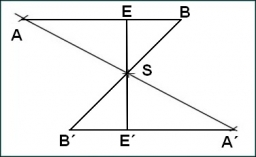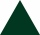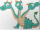# Perimeters

A rectangle has a perimeter of 16p centimeters, it had a width of 2p centimeters. Each side of an equilateral triangle is 1/2 the length of the rectangle. Find the total perimeter of the rectangle and the triangle if p=8.

r =  128 cm
t =  72 cm

### Step-by-step explanation:Did you find an error or inaccuracy? Feel free to write us. Thank you!Tips to related online calculators

#### You need to know the following knowledge to solve this word math problem:

We encourage you to watch this tutorial video on this math problem:

## Related math problems and questions:

• Rectangle 45The perimeter of a rectangle is 60cm. If the length of the rectangle is 20cm. a)find the width b)find the area.
• An equilateral triangleThe perimeter of an equilateral triangle is 33cm. How long is each side?
• The perimeter 3The perimeter of a rectangle is 35 cm. The ratio of the length to its width is 3:2. Calculate the dimensions of the rectangle
• The length 2The length of a rectangle is 12y2 – 15y + 8 and the width is 7y – 11. Find the area of the rectangle
• Second sideCalculate the length of the other side of the rectangle if its circumference is 60 cm and one side is 10 cm long.
• TrianglesEquilateral triangle with side 40 cm has the same perimeter as an isosceles triangle with arm of 45 cm. Calculate the base x of an isosceles triangle.
• The ropeA 68-centimeter long rope is used to make a rhombus on the ground. The distance between a pair of opposite side corners is 16 centimeters. What is the distance between the other two corners?
• Area and perimeter 2Find the area and the perimeter of a rectangle of length 45 1/2 cm and breadth 16 2/3 cm.
• Rectangle from stringString 12m. Make rectangle when one side is two times longer than its width.
• HypotenuseCalculate the length of the hypotenuse of a right triangle if the length of one leg is 4 cm and its content area is 16 square centimeters.
• FloorRectangular floor of living room has a length 5.4 meters and a circumference 17.2 meters. What is its width?
• The circumferenceThe circumference and width of the rectangle are in a ratio of 5: 1. its area is 216cm2. What is its length?
• Perimeter of a rectangleIf the perimeter of a rectangle is 114 meters and the length is twice the width plus 6 meters, what are the length and width?
• The perimeter of the rectangleThe length, l, of a rectangle is 4 inches greater than its width, w. The perimeter of the rectangle is at least 30 inches. What inequality shows the range of possible widths of the rectangle?
• Rectangle 35Find the area of a rectangle when the diagonal is equal to 30 cms and the width is double the length.
• Cuboid - VabFind the surface of the cuboid when its volume is 52.8 cubic centimeters, and the length of its two edges is 2 centimeters and 6 centimeters.Three sides of a quadrilateral are equal to the foreside which is 16 cm long. What is the length of the one with equal sides if the perimeter is 58 cm?